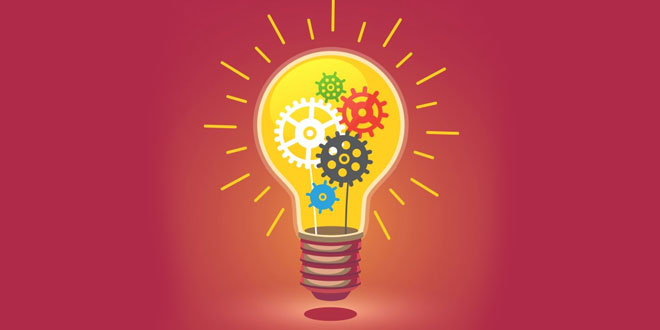Friday , October 7 2022# Electricity: 10th CBSE Science Physics

#### Question: Mention one reason why tungsten is used for making filament of electric lamp.

Answer: It has low resistivity and high melting point.

#### Question: Calculate the number of electrons constituting one coulomb of change. (Charge one electron = 1.6 × 10-19C)

Answer: Number of electron = 1C/1.6 × 10-19C
= 0.625 × 1019
= 6.25 × 10-18

#### Question: (i) Name the instrument / device used to measure electric current in a circuit. (ii) How is an ammeter connected in a circuit to measure current flowing through it?

Answer: (i) Ammeter   (ii) In series

#### Question: In an electric circuit, state the relationship between the direction of conventional current and the direction of flow of electrons.

Answer: The direction of conventional current is opposite to the direction of flow of electrons.

#### Question: How does the resistivity of alloys compare with those of pure metals from which they may have been formed?

Answer: Alloys have higher resistivity in comparison to pure metals.

#### Question: Write SI unit of resistivity.

Answer: Ohm-metre (Ω m).

#### Question: State a difference between the wire used in the element of an electric heater and in a wire.

Answer: The wire used in the element of an electric heater has very high resistance while that in a fuse wire has a low resistance.

#### Question: Power of a lamp is 60 W. Find the energy in joules consumed by it in 1 s.

Answer: Energy consumed = P×t = 60×1 = 60 J

#### Question: Define electrostatic potential at a point.

Answer: Electric potential at a point is defined as the amount of work done in moving a unit charge from infinity to that point.

#### Question: What is the SI unit of electric potential?

Answer: In SI, electric potential is measured in volt (V).

#### Question: Write the mathematical expression for electric potential.

Answer: Mathematically, electric potential is written as V = W/q.

#### Question: Is electrostatic potential a scalar quantity or a vector quantity?

Answer: It is a scalar quantity.

#### Question: What is potential difference? Is it a scalar quantity or a vector quantity? Give its SI units.

Answer: It is the amount of work done in moving a unit charge between two given points. It is a scalar quantity and is measured in volt.

#### Question: When is potential different between two points said to be 1 volt?

Answer: Potential difference between two points is said to be one volt, if a work of 1 joule is done to move a charge of 1 coulomb between the two points.

#### Question: How can we maintain a potential difference across a conductor?

Answer: By using a source of emf. For example, a battery.

#### Question: How many joule are there in one kilowatt hour?

Answer: 3.6 × 10J

#### Question: How can we measure the potential different across the end of a conductor?

Answer: By using a device called voltmeter.

#### Question: How can measure the current in a circuit?

Answer: By using s device called an ammeter.

#### Question: How does the resistance of a wire vary with its cross-sectional area?

Answer: It varies inversely with the cross-sectional area.

#### Question: How is voltmeter connected in a circuit?

Answer: It is always connected in parallel in a circuit.

#### Question: How is an ammeter connected in a circuit?

Answer: It is always connected in series in a circuit.

#### Question: Define current. Give its SI unit.

Answer: Current is defined as the rate of flow electrons. It is measured in ampere (A).

## Sources of Energy: 10th Science Chapter 14

Class: 10th Class Subject: Science Chapter: Chapter 14: Sources of Energy Quiz: – Questions MCQs: …